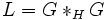# Amalgam-characteristic implies potentially characteristic

Jump to: navigation, search

## Contents

DIRECT: The fact or result stated in this article has a trivial/direct/straightforward proof provided we use the correct definitions of the terms involved
View other results with direct proofs
VIEW FACTS USING THIS: directly | directly or indirectly, upto two steps | directly or indirectly, upto three steps|
VIEW: Survey articles about this
This article gives the statement and possibly, proof, of an implication relation between two subgroup properties. That is, it states that every subgroup satisfying the first subgroup property (i.e., amalgam-characteristic subgroup) must also satisfy the second subgroup property (i.e., potentially characteristic subgroup)
View all subgroup property implications | View all subgroup property non-implications
Get more facts about amalgam-characteristic subgroup|Get more facts about potentially characteristic subgroup

## Statement

Any amalgam-characteristic subgroup of a group is a potentially characteristic subgroup.

## Definitions used

### Amalgam-characteristic subgroup

Further information: Amalgam-characteristic subgroup

A subgroup$H$ of a group$G$ is termed amalgam-characteristic in$G$ if$H$ is a characteristic subgroup in the amalgam$L := G *_H G$.

### Potentially characteristic subgroup

Further information: Potentially characteristic subgroup

A subgroup$H$ of a group$G$ is termed potentially characteristic in$G$ if there exists a group$L$ with an injective map$\alpha: G \to L$ such that the image$\alpha(H)$ is a characteristic subgroup of$K$.

## Related facts

### Converse

The converse is not true. This follows from the fact that characteristic not implies amalgam-characteristic and characteristic implies potentially characteristic. In other words, there are characteristic subgroups that are not amalgam-characteristic. Since any characteristic subgroup is potentially characteristic, we obtain examples of potentially characteristic subgroups that are not amalgam-characteristic.

## Proof

Given: A group$G$ with a subgroup$H$ that is characteristic in the amalgam$L = G*_H G$.

To prove:$H$ is a potentially characteristic subgroup of$G$: there exists a group with an injective map from$G$ to that group such that the image of$H$ is characteristic in that group.

Proof: We claim that the group is, in fact,$L$ itself.

Observe that we can take the injective map$\alpha:G \to L$ as the embedding of the first amalgamated factor$G$. Under this embedding$\alpha(H)$ is the same as the amalgamated$H$, which by assumption is characteristic in$L$. Thus, we have an injective map from$G$ to$L$ under which the image of$H$ is characteristic in$L$.